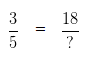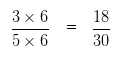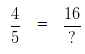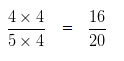# Finding Equivalent Fraction with given Numerator

Question based on Finding an Equivalent Fraction with given Numerator

Question 1

Write an equivalent Fraction of 3/5 with Numerator 18 .

Explanation

Let,In order to obtain an equivalent fraction, we need to multiply the Numerator and Denominator of a given Fraction, by same digit

To get 18 as Numerator, we have to multiply 3 by 6

In order to get an equivalent Fraction, we have to multiply the Denominator by the same number, i.e, 6Hence, 18/30 is an equivalent Fraction of 3/5 with numerator 18.

Question 2

Write an equivalent Fraction of 4/5 with Numerator 16 .

Explanation

Let,In order to obtain an equivalent fraction, we need to multiply the Numerator and Denominator of a given Fraction, by same digit

To get 16 as Numerator, we have to multiply 4 by 4

In order to get an equivalent Fraction, we have to multiply the Denominator by the same number, i.e, 4Hence, 16/20 is an equivalent Fraction of 4/5 with numerator 16.